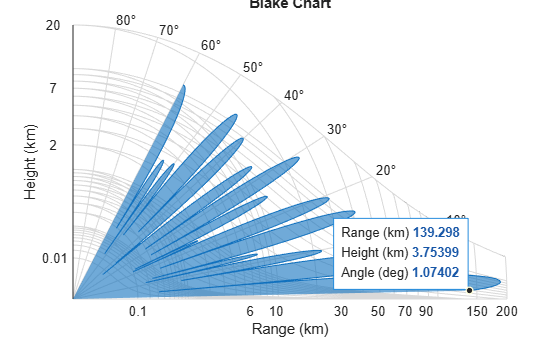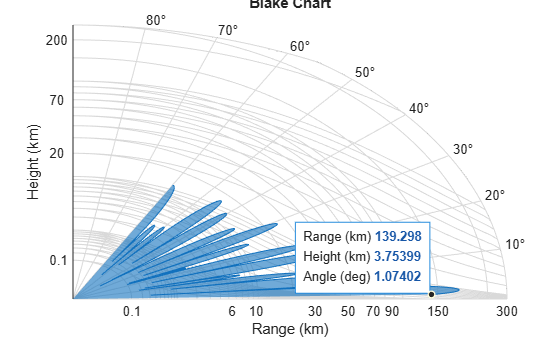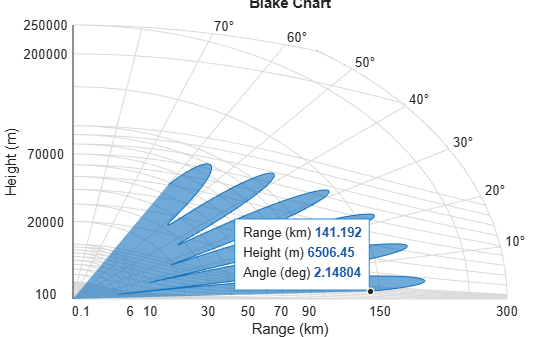# blakechart

Range-angle-height (Blake) chart

## Syntax

``blakechart(vcp,vcpangles)``
``blakechart(vcp,vcpangles,rmax,hmax)``
``blakechart(___,'Name','Value')``

## Description

example

````blakechart(vcp,vcpangles)` creates a range-angle-height plot (also called a Blake chart) for a narrowband radar antenna. This chart shows the maximum radar range as a function of target elevation. In addition, the Blake chart displays lines of constant range and lines of constant height. The input consist of the vertical coverage pattern, `vcp`, and vertical coverage pattern angles, `vcpangles`, produced by `radarvcd`.```

example

````blakechart(vcp,vcpangles,rmax,hmax)`, in addition, specifies the maximum range and height of the Blake chart. You can specify range and height units separately in the Name-Value pairs, `RangeUnit` and `HeightUnit`. This syntax can use any of the input arguments in the previous syntax.```

example

````blakechart(___,'Name','Value')` allows you to specify additional input parameters in the form of Name-Value pairs. You can specify additional name-value pair arguments in any order as (`Name1`,`Value1`,...,`NameN`,`ValueN`). This syntax can use any of the input arguments in the previous syntaxes.```

## Examples

collapse all

Display the vertical coverage diagram of an antenna transmitting at 100 MHz and placed 20 meters above the ground. Set the free-space range to 100 km. Use default plotting parameters.

```freq = 100e6; ant_height = 20; rng_fs = 100; [vcp, vcpangles] = radarvcd(freq,rng_fs,ant_height); blakechart(vcp, vcpangles);```Display the vertical coverage diagram of an antenna transmitting at 100 MHz and placed 20 meters above the ground. Set the free-space range to 100 km. Set the maximum plotting range to 300 km and the maximum plotting height to 250 km.

```freq = 100e6; ant_height = 20; rng_fs = 100; [vcp, vcpangles] = radarvcd(freq,rng_fs,ant_height); rmax = 300; hmax = 250; blakechart(vcp,vcpangles,rmax,hmax)```Plot the range-height-angle curve of a radar having a sinc-function antenna pattern.

Specify antenna pattern

Specify the antenna pattern as a sinc function.

```pat_angles = linspace(-90,90,361)'; pat_u = 1.39157/sind(90/2)*sind(pat_angles); pat = sinc(pat_u/pi);```

Set the transmitting frequency to 100 MHz, the free-space range to 100 km, the antenna tilt angle to ${0}^{\circ }$, and place the antenna 20 meters above the ground. Assume a surface roughness of one meter.

```freq = 100e6; ant_height = 10; rng_fs = 100; tilt_ang = 0; surf_roughness = 1;```

```[vcp, vcpangles] = radarvcd(freq,rng_fs,ant_height,... 'RangeUnit','km','HeightUnit','m',... 'AntennaPattern',pat,... 'PatternAngles',pat_angles,'TiltAngle',tilt_ang,... 'SurfaceRoughness',surf_roughness);```

Set the maximum plotting range to 300 km and the maximum plotting height to 250,000 m. Choose the range units as kilometers, `'km'`, and the height units as meters, `'m'`. Set the range and height axes scale powers to 1/2.

```rmax = 300; hmax = 250e3; blakechart(vcp, vcpangles, rmax, hmax, 'RangeUnit','km',... 'ScalePower',1/2,'HeightUnit','m');```## Input Arguments

collapse all

Vertical coverage pattern specified as a K-by-1 column vector. The vertical coverage pattern is the actual maximum range of the radar. Each entry of the vertical coverage pattern corresponds to one of the angles specified in `vcpangles`. Values are expressed in kilometers unless you change the unit of measure using the `'RangeUnit'` Name-Value pair.

Example: [282.3831; 291.0502; 299.4252]

Data Types: `double`

Vertical coverage pattern angles specified as a K-by-1 column vector. The set of angles range from –90° to 90°.

Example: [2.1480; 2.2340; 2.3199]

Data Types: `double`

Maximum range of plot specified as a real-valued scalar. Range units are specified by the `RangeUnit` Name-Value pair.

Example: 200

Data Types: `double`

Maximum height of plot specified as a real-valued scalar. Height units are specified by the `HeightUnit` Name-Value pair.

Example: 100000

Data Types: `double`

### Name-Value Pair Arguments

Specify optional comma-separated pairs of `Name,Value` arguments. `Name` is the argument name and `Value` is the corresponding value. `Name` must appear inside quotes. You can specify several name and value pair arguments in any order as `Name1,Value1,...,NameN,ValueN`.

Example: ‘RangeUnit’,’m’

Range units denoting nautical miles, miles, kilometers, feet or meters. This Name-Value pair specifies the units for the vertical coverage pattern input argument, `vcp`, and the maximum range input argument, `rmax`.

Example: `'mi'`

Data Types: `char`

Height units specified as one of `'nmi'` | `'mi'` | `'km'` | `'ft'` |`'m'` denoting nautical miles, miles, kilometers, feet or meters. This Name-Value pair specifies the units for the maximum height, `hmax`.

Example: `'m'`

Data Types: `char`

Scale power, specified as a scalar between 0 and 1. This parameter specifies the range and height axis scale power.

Example: 0.5

Data Types: `double`

Surface refractivity, specified as a non-negative real-valued scalar. The surface refractivity is a parameter of the CRPL Exponential Reference Atmosphere Model used in this function.

Example: 314

Data Types: `double`

Refraction exponent specified as a non-negative, real-valued scalar. The refraction exponent is a parameter of the CRPL Exponential Reference Atmosphere Model used in this function.

Example: 0.15

Data Types: `double`

collapse all

### CRPL Exponential Reference Atmosphere Model

The `blakechart` function uses the CRPL Exponential Reference Atmosphere to model refraction effects. The index of refraction is a function of height

`$n\left(h\right)=1.0+\left({N}_{s}\text{\hspace{0.17em}}×\text{\hspace{0.17em}}{10}^{-6}\right){e}^{-{R}_{exp}h}$`

where Ns is the atmospheric refractivity value (in units of 10–6) at the surface of the earth, Rexp is a decay constant, and h is the height above the surface in kilometers. The default value of Ns is 313 and can be modified using the `'SurfaceRefractivity'` Name-Value pair. The default value of Rexp is 0.143859 and can be modified using the `'RefractionExponent'` Name-Value pair.

 Blake, L.V. Machine Plotting of Radar Vertical-Plane Coverage Diagrams. Naval Research Laboratory Report 7098, 1970.